StatLect

# Sign of a permutation

This lecture introduces the concept of sign (or signature) of a permutation of a set of natural numbers. The concept will be used in the definition of the determinant of a matrix.## Permutations of the first n natural numbers

We are going to assume that the reader is already familiar with the concept of permutation.

We are going to deal with permutations of the set of the firstnatural numbersRemember that a permutation is one of the possible ways to order the elements of a set.

We will denote a permutation by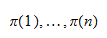whereis the first element of the permutation,is the second, and so on.

Example Consider the set of the first four natural numbersThen,is a permutation. Its elements can be denoted as follows: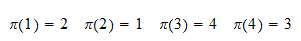Another permutation isand we write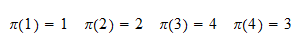Also remember that the number of all possible permutations of the firstnatural numbers is the factorial of: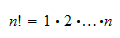Example There arepossible permutations of the first three natural numbers. They are## Inversions

An important concept is that of inversion.

Definition A couple of elements of a permutationandis said to be an inversion if and only ifbutIn other words, two elements are an inversion if their natural order is inverted.

Example Consider the following permutation of the first five natural numbers:It contains the following inversions:## Parity of a permutation

We now define the parity of a permutation.

Definition A permutation is said to be even if and only if the total number of inversions it contains is even. Otherwise, it is said to be odd.

In the previous example there wereinversions. So, the parity of the permutation in that example was odd.

Here is another example.

Example Consider the following permutation of the first four natural numbers:Its inversions areThus, there are a total ofinversions. As a consequence, the permutation is even.

## Definition of sign

We are now ready to define the concept of sign (or signature).

Definition The sign of a permutation, denoted byis a function defined as follows: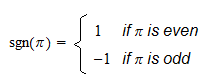Example The permutation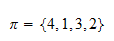defined in the previous example was even. Therefore,.

## Transpositions

We are now going to analyze how simple changes in the order of the elements of a permutation affect its sign.

Definition The operation of interchanging any two distinct elements of a permutation is called a transposition. If the elements are adjacent, then it is called an adjacent transposition.

Example Consider the following permutation of the first five natural numbers:The operation of interchanging its second and fourth element so as to obtain the new permutationis a transposition. If we now interchange the first and second elementwe are performing an adjacent transposition.

The effect of transpositions on parity is characterized as follows.

Proposition If a permutation is odd, a transposition makes it even.

Proposition If a permutation is even, a transposition makes it odd.

Proof

Let us start from the simpler case of an adjacent transposition. Letandbe the two elements involved in the transposition. Ifandare not an inversion, then they become an inversion. No other inversions are affected by the transposition. As a consequence, the total number of inversions in the permutation increases by one unit. On the contrary, ifandare an inversion, then by interchanging them we remove the inversion and the total number of inversions decreases by one unit. Thus, the number of inversions either decreases or increases by one unit. If it is odd, it becomes even. If it is even, it becomes odd. In other words, the two propositions above hold for adjacent transpositions. Let us now tackle the case in which the transposition is not adjacent. Letandbe two elements involved in the transposition. We performadjacent transpositions to moveto position(we always interchangewith the nearest element on its right). After performing theseadjacent transpositions,is in the right position (), whileis in position. We now performadjacent transpositions to moveto position. Therefore, we perform an odd number () of adjacent transpositions. Since each adjacent transposition changes the parity of the permutation, an odd number of them changes the parity (even becomes odd and vice-versa).

## Solved exercises

Below you can find some exercises with explained solutions.

### Exercise 1

Find the number of inversions in the permutationSolution

The inversions areSo, there areinversions.

### Exercise 2

Determine the sign of the permutationSolution

The inversions areSo there areinversions, the parity is odd, and the sign of the permutation is.

### Exercise 3

Consider the permutation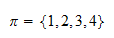and the transposition of its first and fourth element, giving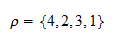Write down a sequence of adjacent transpositions that allows you to obtain the same result.

Solution

We first move the first element to the last position by adjacent transpositions:The elementis now in the penultimate position and we move it backwards: